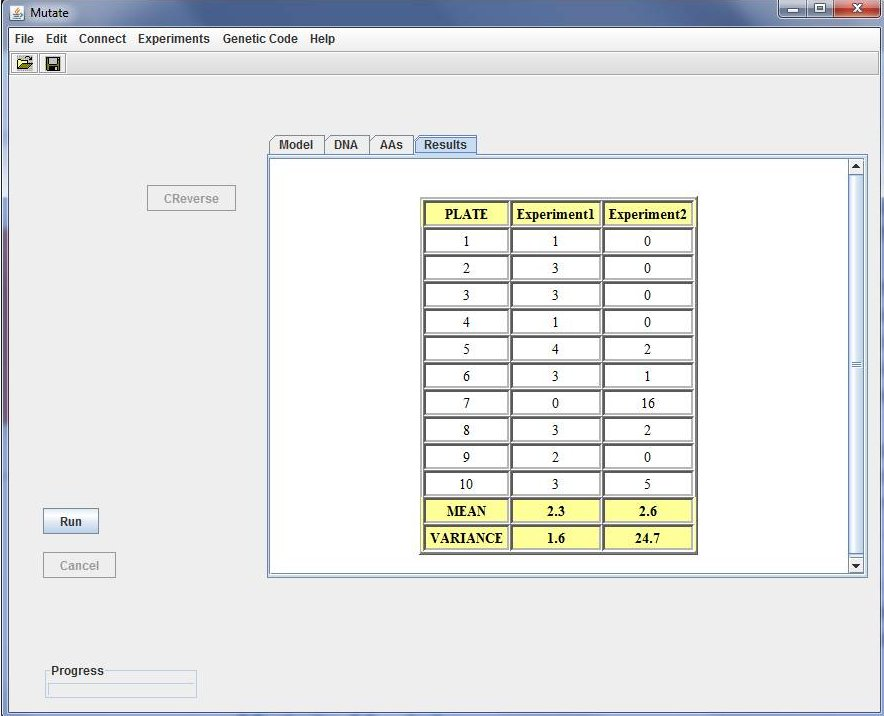MUTATE: IN SILICO PERFORMANCE OF THE FLUCTUATION TESTHints
1. After download Mutate requires the Java 5.0 (or higher) Runtime Environment freely available at http://www.java.com.
2. Whatever the operating system, you should be able to run the program just by double clicking on the mutate.jar file
3. WINDOWS USERS: If double-clicking the .jar file does not open the program, make sure that the jar extension is associated with javaw.exe (in your JRE folder).

Contact
For any questions you can contact me

Example

``````

a) E1: N = 106, µ = 10-8, P = 10. E2: N = 105, µ = 10-8, P = 10.

b) E1: N = 107, µ = 10-8, P = 10. E2: N = 106, µ = 10-8, P = 10.

c) E1: N = 108, µ = 10-8, P = 10. E2: N = 107, µ = 10-8, P = 10.

d) E1: N = 109, µ = 10-8, P = 10. E2: N = 108, µ = 10-8, P = 10.

e) 10E1: N = 109, µ = 10-8, P = 10. 10E2: N = 108, µ = 10-8, P = 10.

f) 100E1: N = 109, µ = 10-8, P = 10. 100E2: N = 108, µ = 10-8, P = 10.

g) 200E1: N = 109, µ = 10-8, P = 10. 200E2: N = 108, µ = 10-8, P = 10.

h) E1: N = 108, µ = 10-8, P = 5. E2: N = 107, µ = 10-8, P = 5.

i) E1: N = 108, µ = 10-8, P = 50. E2: N = 107, µ = 10-8, P = 50.

``````

Explain the obtained results. What hypothesis, adaptative or preadaptative, is better supported by the data?. What happens when increasing the number P of plates and/or the experiment replicates?. Please, note that the number appearing before the experiment identifier i.e. as in 10E1, refers to the number of experiment repetitions or replicates. That is, E1 stands for the results of performing the experiment-1 just one time while 10E1 refers to results averaged through 10 experiment replicates. Assuming that mutation ocurrence follows a Poisson distribution, estimate the mutation rate µ from the E2 experiment of case d) as

``````
µ = m / (Nt - N0).
``````

Note that the expected number of mutations m that occurred per plate can be computed from the proportion p of cultures without mutants, being

``````
p = e-m
``````

A. Carvajal-Rodriguez - Departamento de Bioquímica Genética e Inmunología - Universidad de Vigo. ( Last update: December 2011)